# ARR – Accounting Rate of Return

A corporate finance statistic that can be used to calculate the expected percentage rate of return

Investing in long-term assets involves careful structural planning, a budget, and the conviction that investing in the asset will yield a substantial reward in the future.The accounting rate of return is a corporate finance statistic that can be used to calculate the expected percentage rate of return on a capital asset based on its initial investment cost.

ARR helps businesses decide which assets to invest in for long-term growth by comparing them with the return of the other assets.

ARR is also known as the simple rate of return because it doesn't take into account the concept of the time value of money, which states that the present value of money is worth more now than in the future.

ARR comes in handy when the investment needs to be evaluated based on the profits rather than the cash flow it expects to generate in the future.

This is one of the simplest methods to calculate the return on any investment, so sometimes, it acts as a tool for the short-term investor to segregate various investment opportunities and focus on those who remain within the required rate of return of the investor.

The formula for calculating the Accounting Rate of Return is-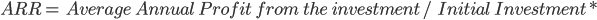## Note

Instead of initial investment, we can also take average investments, but the final answer may vary depending on that.

Steps for calculating ARR

1. Calculate the total net profit from the investment, which includes revenue generated by the asset, excluding all the necessary expenses incurred, such as operating expenses, interest, taxes, etc.

2. After calculating the total net profit arising from the asset, divide it by its estimated working life to arrive at the average annual profit from the investment.

If the investment is in the fixed asset, then work out the depreciation aspect to be deducted at the end while calculating the net annual profit of the asset.

3. Finally, divide the Average Net annual Profit from the investment by the initial investment to arrive at the Accounting Rate of Return.

## Examples for calculation of Accounting Rate of Return

Example #1

A company decided to purchase a fixed asset costing \$25,000.This fixed asset would help the company increase its revenue by \$10,000, and it would incur around \$1,000.The useful life of the fixed asset is 20 years. What would be the Accounting Rate of Return for the year?

Since it is about the fixed asset, we need to take into account the amount of depreciation to calculate the annual net profit of the required investment.

So,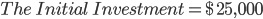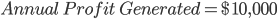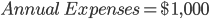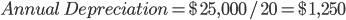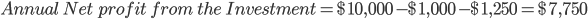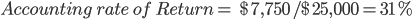This 31% means that the company will receive around 31 cents for every dollar it invests in that fixed asset.

Example #2

ABC Company is considering investing in a country to expand its business. For that, they require an investment of around \$250,000.The total Cash Inflow from the investment would be around \$50,000 in the 1st Year, \$45,000 for the next three years, and \$30,000 for the 5th year. In the end, the investment will have a salvage value of around \$50,000.

So, the first step would be to calculate the average annual profit.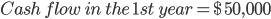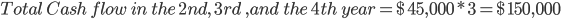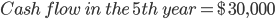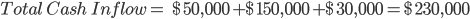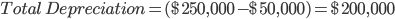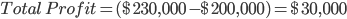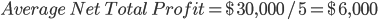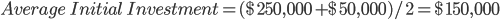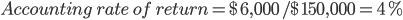In this example, there is a 4% ARR, meaning the company will receive around 4 cents for every dollar it invests in that fixed asset.

## Calculation of ARR in excel

It is crucial to record the return on your investment using programs like Microsoft Excel or Google Sheets to keep track of it. If you are using excel as a tool to calculate ARR, here are some of the most important steps that you can take.

Year 12345
Net Income 10,00015,00020,00025,00030,000
Initial Investment100,000
Salvage Value20,000
ARR33%

1. Firstly mention the year in the first cell of excel, and then corresponding to that, mention the number of years you want the project to give the returns. In the above example, we have assumed five years.

2. Write down the initial investment amount in the second row here. It is 1,00,000, which acts as the major cash outflow for the project.

3. Mention salvage value, if any, in the table (here, we have assumed it to be around 20,000).

4. Now mention ARR in the last cell and put a formula in the cell with an average of all the net income values mentioned in a row divided by the average of initial investment, which is 1,00,000 and 20,000, arrive at the percentage figure of ARR. In the above example, it is 33.3% which means for every dollar the company invests, and it will receive around 0.33 cents in return.

There are various advantages and disadvantages of using ARR when evaluating investment decisions.

• It is one of the simplest methods to calculate the profitability of a project.

• It is easy to calculate and understand the payback pattern of the required investment.

• It is useful compared with other companies and helps us know which company is performing well against the others.

• Short-term investors use this method more frequently for assessing their investment decisions.

• It helps in evaluating the current performance of an investment.

• It displays the investment's cumulative book profit so that it can be contrasted with the results of other initiatives.

• Useful for demonstrating how well a project performs over time.

• It can be used to determine the projects a firm should invest in and those that it shouldn't.

• It can help in determining how well the project does in terms of profits rather than cash flows.• One of the main disadvantages of this method is that it ignores the time value of money, which is the most important concept for determining the overall investment opportunity.

• This method of calculating profit cannot be used when the investment is being made on an installment basis rather than lump-sum.

• This method ignores the factors that are external to the business environment of the company, which affects the investment decision of the firm.

• While calculating the return from the R.O.I. method, the answer will be different from that of the A.R.R. R.O.I. method is the most suitable method for calculating the project's return when the investment is long-term in nature; hence A.R.R. is not suitable when the investment is for long term nature.

• This method is based only on accounting profits and does not make adjustments such as cash flows, taxes, etc.

• This project does not consider the period of the various investment; hence it may not produce accurate and desired results when comparing it with the other investments.

• Because the Accounting Rate of Return is a metric that represents the total investment and can be distorted by changes in the beginning book value, smaller businesses may not have a strong A.R.R.

• Don't factor in potential savings or future expenditures associated with investments.

• Regarding the initial financial outlay, this method does not account for dividends or any other sources of funding.

• Accounting rates of return may be skewed if only cash in and cash out are considered, rather than how much it will cost to replace assets when they wear out.

## Difference between ARR and IRR

ARR versus IRR
Basis of DifferenceAccounting Rate of Return (A.R.R)Internal Rate of Return (I.R.R)
MeaningIt is the expected amount of profit a project can generate if invested in it.It is the yield percentage that a project is expected to provide over its useful life and is heavily dependent on the cash flow.
FormulaARR is calculated by taking the average annual profit generated from the initial investment and dividing it by the initial amount of investment.IRR is the rate at which the difference between the net present value of future cash flow ie NPV and the cash outflow is zero.
ComplexityIt is easy and simple to calculate.It is much more complex than ARR cause it requires an algebraic formula.
Profits and Cash FlowARR requires the amount of profit being generated from the initial investment.IRR needs cash flow to decide the overall return from the initial investment.
Time Value of MoneyARR does not consider the time value of money while calculating, which forms the essential part of the overall investing decisionIRR does consider the time value of money as it involves discounting all cash flows to their present value
Weightage to the timing of profit and cash flow generationThe ARR method gives equal weightage to the profit across all the years.The IRR method gives more weightage to the cash flows of the current year.

## How is RRR different from ARR?

ARR is the annual percentage of profit or returns received from the initial investment, whereas RRR is the required rate of return that the investor wants. RRR is also known as the hurdle rate.RRR can be calculated using the dividend discount model or CAPM (Capital Asset Pricing Model).

ARR is constant, but RRR varies across investors because each investor has a different variance in risk-taking.

For example, if a project has an ARR of 20% and an investor thinks that the company would outgrow itself, then he would settle for a less expected return in the short run, but the investor who takes calculated risk then would demand a high percentage of return for his investment.

Difference between ARR and Profitability Index

ARR versus Profitability Index
Basis of DifferenceAccounting Rate of ReturnProfitability Index
MeaningIt is the amount of return in terms of the percentage of the initial investment that an investor gets after investing the asset.The future cash inflow's present value divided by the number of cash outflows
Focuscentered on calculating the % returns on an investmentHow many times the initial investment will be recovered is the main goal of PI.
Expressed asIt is expressed in the form of a percentage(%)It is expressed in the form of the ratio
Discount RateFinding a suitable discount rate is not a challenge for ARR.Uses the discount rate to discount future cash flows.
Calculation of Present ValueThe present value of future cash flows is not determined by ARRThe present value of future cash flows is determined using the PI technique.### Everything You Need To Build Your Accounting Skills

To Help You Thrive in the Most Flexible Job in the World.

Researched and authored by Ashish Sharma I LinkedIn